# Civil Engineering - UPSC Civil Service Exam Questions

16.

A simply supported beam of span 'l' is loaded (as shown in the given figure) with a uniformly varying load of intensity w1/unit length at 'A' to w2/unit length at 'B'. A The shear force at the support 'B' is given by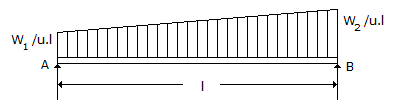A.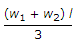B.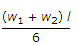C.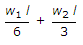D.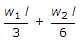Explanation:

No answer description available for this question. Let us discuss.

17.

In the PERT analysis, which one of the following is followed by the time estimate of activities and probability of either occurrance ?

 A. Normal distribution B. Poisson's distribution C. β - distribution D. Binomial distribution.

Explanation:

No answer description available for this question. Let us discuss.

18.

The cable for a prestressed concrete simply supported beam subjected to uniformly distributed load over the entire span should ideally be :

 A. placed at the centre of cross-section over the entire span B. placed at some eccentricity over the entire span C. varying linearly from the centre of cross-section at the ends to maximum eccentricity at the middle section D. parabolic with zero eccentricity at the ends and maximum eccentricity at the center of the span

Explanation:

No answer description available for this question. Let us discuss.

19.

The effort 'P' to be applied horizontally to pull a weight 'W' on a plane inclined at an angle α with the horizontal is given by (tan θ is the coefficient of friction)

 A. P = W tan (α + θ) B. P = W tan (α - θ) C.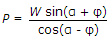D.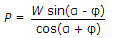Explanation:

No answer description available for this question. Let us discuss.

20.

If two triangulation signals of 6.75 m height each, are to be just visible over ground mutually, what is the maximum distance between their locations on the ground surface ?

 A. 10 km B. 20 km C. 30 km D. 40 km